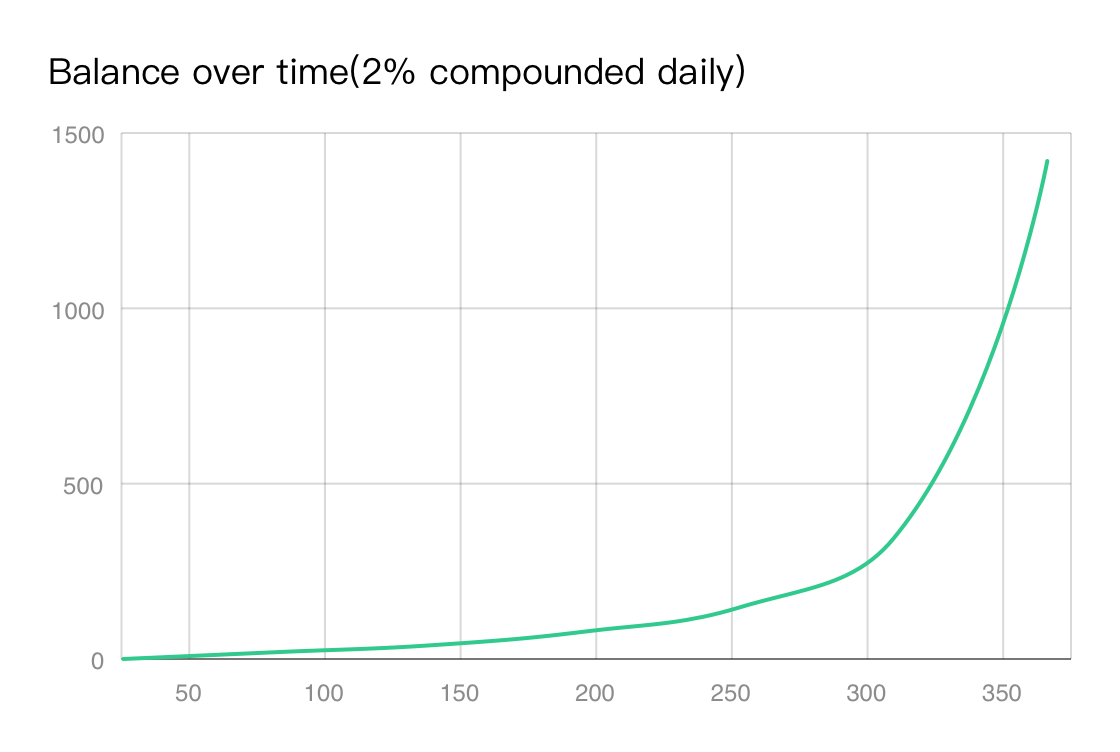What is APY
APY stands for Annual Percentage Yield. It measures the real rate of return on your principal by taking into account the effect of compounding. In the case of XenophonDAO, the XPH you stake represents your principal and, due to the rebase mechanism, compound interest is added periodically at each period (approximately 8 hours).
The APY is calculated based on the rewardYield (aka rebase rate) using the following equation.
$APY = (1 + rewardYield)^{365*3}$
Rebase occurs 3 times a day, 365 days a year, which would give a rebase frequency of 365 * 3 = 1,095.
The rewardYield calculation formula is as follows:
$rewardYield = XPHdistributed/XPHtotalStaked$
The amount of XPH allocated to the Staking contract is calculated from the total XPH supply using the following equation:
$XPHdistributed =XPHtotalSupply*rewardRate$
An interesting fact about APY is that your balance grows exponentially! Assuming a 2% compounding rate per day, if you start with a balance of 1 XPH on day one, your balance will grow to about 1,377 XPH after one year.Assuming you have \$1,000 and two investment options, one earning 2% simple interest per day and the other earning 1.5% compound interest per day, the comparing results are as follows:
Over the course of a week, simple interest would net 14% (0.02 * 7), while compound interest would net 11% (1.015⁷-1); Over the course of a month, simple interest would net 60%, while compound interest would net 56%; Over the course of a year, simple interest would net 730% while compound interest would net 22,814%.
This is the reason why XenophonDAO protocol attracts so many users.